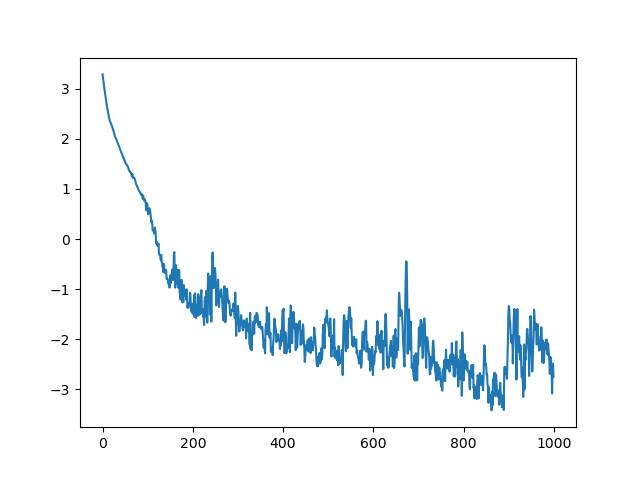# Log_prob yields positive values during training

I tried to use normalizing flows to transfer some distribution to the normal distribution. As a transform I used

``````arn = AutoRegressiveNN(2, , param_dims=[count_bins,count_bins,count_bins-1,count_bins])
spline = SplineAutoregressive(2, arn, order='linear', count_bins=count_bins)
base_dist = dist.Normal(torch.zeros(2), torch.ones(2)) flow_dist = dist.TransformedDistribution(base_dist, [spline])
``````

However, during training the `- flow_dist.log_prob(x).mean()` command decreased below zero and did not stop decreasing as depicted in the following figure.I would have expected that `- flow_dist.log_prob(x).mean()` is strictly greater zero for all inputs. Is this an issue?

Below you find the full MWE. Thanks for any help.

``````import torch
import numpy as np
from tqdm import tqdm
import pylab as plt
import pyro.distributions as dist
from pyro.nn import AutoRegressiveNN
from pyro.distributions.transforms import SplineAutoregressive

flow_layers = 0
count_bins = 8

arn = AutoRegressiveNN(2, , param_dims=[count_bins,count_bins,count_bins-1,count_bins])
spline = SplineAutoregressive(2, arn, order='linear', count_bins=count_bins)

base_dist = dist.Normal(torch.zeros(2), torch.ones(2))
flow_dist = dist.TransformedDistribution(base_dist, [spline])

vals = np.zeros((1000, 1))

x = torch.Tensor([[1, 0],
[0, 1],
[-1, 1],
[1, 1],
[1, -1],
])

pbar = tqdm(range(vals.shape))
for i in pbar:

prob = - flow_dist.log_prob(x).mean()
vals[i] = prob.item()

pbar.set_postfix(prob=prob.item())

prob.backward()
optimizer.step()
flow_dist.clear_cache()

Y = flow_dist.sample(torch.Size([1000,]))
plt.figure()
plt.scatter(Y[:,0], Y[:,1], color='firebrick', label='flow', alpha=0.5)

plt.figure()
plt.plot(vals)

plt.show()
``````

UPDATE:

May be it is already a problem in PyTorch. Executing the following code

``````import torch
from torch import distributions

dist = distributions.Normal(torch.Tensor([0.]),torch.Tensor([0.1]))
print(dist.log_prob(torch.Tensor([0.15])))
``````

results is ` tensor([0.2586])`, which should be a negative number. Is this an issue or do I do something wrong?

UPDATE:
I found this post, which clarifies that log_prob is the log of the density function.

Thanks for asking on the forum! Just to clearly answer: `my_dist.log_prob(x)` is the log of the density function. While the density must be nonnegative, its log can be any real number.

1 Like